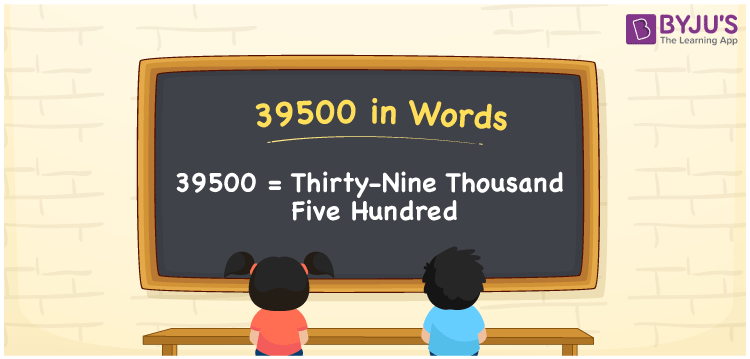# 39500 in Words

Thirty-nine Thousand Five Hundred is the word for the number 39500. Using the place value of a given number, the number 39500 can be easily converted to words. As a result, the number names must be created using a place value table. For instance, if you purchased a laptop that costs Rs. 39500, you can write “I purchased a laptop that costs Rs. Thirty-nine Thousand Five Hundred”. In this article, we’ll learn how to spell 39500 in English and how to express the number 39500 in words using a place value chart.

 39500 in Words: Thirty-nine Thousand Five Hundred. Thirty-nine Thousand Five Hundred in Numerical Form: 39500.

## 39500 in English Words## How to Write 39500 in Words?

A place value chart can be used to convert numbers to words. The place value chart for the number 39500 is shown below.

 Ten-thousands Thousands Hundreds Tens Ones 3 9 5 0 0

The expanded format of the number 39500 is presented below.

= 3 × Ten thousand + 9 × Thousand + 5 × Hundred + 0 × Ten + 0 × One

= 3 × 10000 + 9 × 1000 + 5 × 100

= 30000 + 9000 + 500

= 39500

= thirty-nine thousand five hundred

Hence, 39500 in words is Thirty-nine thousand five hundred.

After 39499 and before 39501, the natural number 39500 appears.

39500 in words – Thirty-nine thousand five hundred

Is 39500 an odd number? – No

Is 39500 an even number? – Yes

Is 39500 a perfect square number? – No

Is 39500 a perfect cube number? – No

Is 39500 a prime number? – No

Is 39500 a composite number? – Yes

## Frequently Asked Questions on 39500 in Words

Q1

### How to write 39500 in words?

39500 in words is thirty-nine thousand five hundred.

Q2

### Simplify 39000 + 500, and express in words.

Simplifying 39000 + 500, we get 39500. Hence, 39500 in words is thirty-nine thousand five hundred.

Q3

### Convert thirty-nine thousand five hundred into numbers.

Thirty-nine thousand five hundred in numbers is 39500.